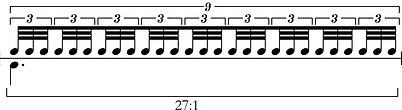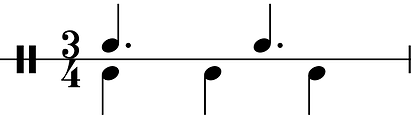top of page# Overview

The purpose of this talk is to outline and explore the fundamental principles of polyrhythms, and the concept of polyrhythmic music in general. The main topics in this talk are:

• The basics of polyrhythms

• Tuplets as polyrhythms

• An introduction to polyrhythmics

# I. Polyrhythms

The concept of polyrhythms is somewhat difficult to define. Many musicologists have presented technically correct and accurate definitions that regularly fail to convey any sense of explanation. Bob Becker, in his seminal Rudimental Arithmetic, defines polyrhythms as follows:

An interrelationship of simultaneous groups of beats and/or groups of pulses that can be expressed by a ratio with two or more factors not equal to 1. (p.12)

From a technical standpoint, this is an excellent definition, but it is unlikely to clarify the issue for new students. We can clarify, however, by exploring the terms within this definition. We established in previous episodes how rhythms are created by the relationship between subdivision and time signature, in short: meter. This relationship can be defined by a ratio, corresponding to the subdivision and the beat respectively. Within the time signature of 4/4, a four-note subdivision of 16th notes per quarter note would be described as a 4:1 ratio: four 16th notes to every one quarter note.

By this concept, 8th notes in a ternary time signature hold a ratio of 3:1 against the dotted quarter note pulse, while a 16th note sextuplet in binary time would be described as 6:1. By using tuplets, more complex subdivision ratios can be established, depending on the meter.

For example, establishing a ratio of 27:1 against a dotted quarter note requires 32nd note triplets tested within 16th note nonuplets. Larger tuplets, like 8th note quintuplets, would hold a ratio of 2.5:1 against a quarter note, as only half of the tuplet is completed within the space of each quarter note.

With this established, we can return to Becker’s definition, which speaks of simultaneous subdivisions expressed by a ration with two or more factors not equal to 1. We are now in a position to explore what this means.

Polyrhythms can themselves be expressed by a ratio in the same way as subdivisions. Becker’s definition states that a polyrhythm must have two or more factors within this ratio not equal to 1. The smallest possible polyrhythm, therefore, can be expressed as 2:3, comprising the two smallest prime numbers, and the first two numbers greater than one.

As we discussed with regard to tuplets, which we shall revisit shortly, this can be thought of as two in the space of three. For every two x, there will be three y, and vice versa. Crucially, this relationship implies the existence of a common beat. For simple cross rhythms, the beat will correspond to one of the factors in a two-part ratio, while larger factor polyrhythms will contain a third (or higher) factor corresponding to the metric beat.

Sticking with the 3:2 cross-rhythm for now, we can explore what this means. The nature of a polyrhythm depends on the notion of resolution, which describes the point at which the whole sequence restarts and repeats. This is the total cyclic duration of the polyrhythm. Each factor within the polyrhythm requires its own resolution point, which align in some way to produce the polyrhythm.

With a two-factor polyrhythm like 3:2, we can begin with the resolution point of either factor. Taking the three as this point, we can quite simply play three evenly spaced strokes, corresponding to this factor of the polyrhythm.

The tempo of these three strokes creates a duration, which defines the resolution point of this factor. Now, against this duration, we must also play two strokes of equal duration, which resolve within the same duration of the three. Remember that 3:2 describes three in the space of two (or vice versa), so the two evenly spaced notes of the 2 must occur within the same duration as the three evenly spaced notes of the three.

At this point, the note values involved do not matter; we have to concurrent rhythms occurring with a ratio with factors not equal to 1. If we wish to put this into a metric context, we can write this particular polyrhythm in a time signature of 3/4. In this metric context, we can begin by plotting the three notes of the 3 as the three quarter notes of the bar. This defines the resolution point of the polyrhythm: the three quarter notes occur within the space of one bar of 3/4. Against this, we must plot the two evenly spaced notes of the 2, occupying the same total duration. In other words, against these three quarter notes, we need to place two evenly spaced notes which equate to three quarter notes. Within this context, the only way to achieve this is to use two dotted quarter notes.

Recall that quarter notes comprise two 8th notes each, and so three of them in a bar of 3/4 equate to six 8th notes. Likewise, dotted quarter notes comprise three 8th notes, and so two of them also equate to six 8th notes. We can therefore conceive of our polyrhythm as existing on an 8th note grid, with the binary and ternary groupings occurring simultaneously.

Polyrhythms can exist with factor ratios of many different kinds, so long as they are not reducible. For instance, we could note define a polyrhythm of 6:4 when we could better describe it as two instances of 3:2. However, as Becker describes in his work, there are drum-specific reasons why a 6:4 might better describe the musical idea. This is beyond the scope of this video.

Higher order polyrhythms simply include additional factors. While exceedingly rare and practically difficult to perform, they are nevertheless possible within the theory, and often exist within ensemble context. The same rules apply, such that none of the three factors must be equal to 1, and none must be reducible. For instance, a three-factor polyrhythm might be 5:3:2, in which an even grouping of 5, occur evenly against an even group of three, which both occur simultaneously against an even group of three. It goes without saying that the higher factors of the ratio occur more frequently – faster – than the lower factors.

To work out such polyrhythm, the factors must be multiplied together to create the lowest common multiple. In this case, 5 x 3 x 2 = 30, meaning 30 notes are required to ploy one cycle of the polyrhythm. On these 30 notes, each factor occurs relative to its divisibility by 30. 30 divided by 5 is 6, and so the factor of 5 is placed every six notes. 30 divided by 3 is 10, so the factor of 3 is placed every 10 notes, while the factor of 2 is placed every 15 notes. To play this, we would simply count to 30, and play each factor as it occurs.

# II. Tuplets

Previously, I have stated that tuplets are themselves a specific type of subdivision, and this should now be becoming clearer based on the polyrhythm ratios. If we consider a simple 16th note quintuplet, this would have the ratio of 5:4, read as five 16th notes in the space of four. It now becomes clear that this tuplet implies a 5:4 polyrhythm against the underling quarter note. There is a 5:4 polyrhythm occurring each quarter note, except the factor of 4 is implied by the native 16th note subdivision. In this way, we can describe tuplets as discrete polyrhythms.

# III. Polyrhythmics

To close, I want to briefly explore the concept of polyrhythmics. Music may be polyrhythmic without explicitly expressing full polyrhythms. A polyrhythm can be conceived of as a running train, onto which we can hop and hop off again, without riding it to its final destination.

Taking as our example a 5:4 polyrhythm, describing a factor of five notes occurring concurrently against a factor of four, we can create this by playing accented 16th notes in the time signature of 5/4. The time signature established the factor of 5, with five quarter notes occurring throughout one bar. If we play 16th note against this and accent every 5th stroke, this will produce four evenly spaced accents against the five evenly spaced quarter notes. In terms of the resolution point, this cycle takes on bar of 5/4. However, if we import this into the time signature of 4/4, with only four quarter notes per bar, the whole polyrhythm requires five bars to resolve.

In polyrhythmic music, we can play some of this polyrhythm without having to ride it all the way to resolution. We can hear instances of the 5:4 accent pattern occurring within the larger rhythmic phrasing. In this way, music can be polyrhythmic, without always having to voice entire polyrhythms to resolution.A 27:1 subdivision, comprising 32nd note tuplets nested within a 16th note nonupletTwo instances of a 3:2 polyrhythm, firstly using note values, and secondly using accentsA 5:2 polyrhythm consisting of an 8th note quintuplet over quarter notes, with a 2.5:1 ratio against the beatA 5:4 polyrhythm comprising four evenly spaced accents over five beatsA 5:3:2, three-factor polyrhythm, plotted on a 30-note grid

bottom of page